Simple Elastic Rod Model for DNA Supercoiling

Umut Eser October 31, 2007

(Submitted as coursework for Physics 210, Stanford University, Autumn 2007)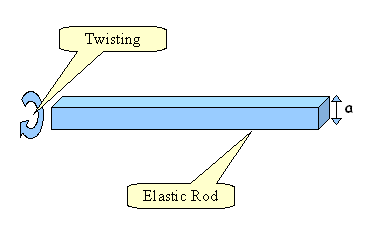Fig. 1: The elastic rod model.

DNA transcription has a vital role in an animate being. But the complexity in the system does not let us to understand the dynamics precisely. In order to understand the mechanisms of transcription, the supercoiling phenomena must be unveiled. Therefore, we investigate the role of elasticity in supercoiling of DNA by making a model which has a similar dynamics.

Before going into the detail of the model, we should introduce some topological concepts that have been used among the researchers of this field. The number of helical turns along the axis of a DNA is called the twist (Tw)(Fig. 1). If one twists an elastic cord, it's longitudinal axis starts wounding around itself to release the torque (Fig. 2). This topological quantity is called writhe (Wr) of the molecule. For a DNA, the total number of helix cross (of two strands) by twist and by writhe is a topological invariant as long as it constrained torsionally. This number is called the linking number Lk = Tw + Wr . The linking number is same as the twist if the DNA is relaxed and linear, i.e. Lk = Lk0 = Tw0 . And until a certain twist number, no writhe can be observed.

To find out when a writhe occurs if we keep twisting the DNA, lets consider an arc with an angle θ from an elastic rod which has a square cross section a2 and the Young Modulus E (Fig. 3). The length of the arc is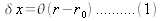If we consider it as a spring, the spring constant of an infinitesimal layer at radius r would be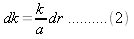where k is the spring constant of the arc, and given by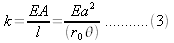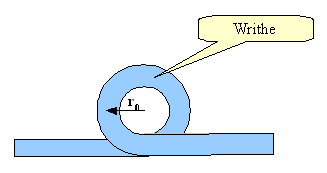Fig. 2: A writhe with a radius r.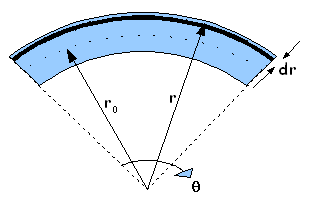Fig. 3: A writhe with a radius r.

Then the bending energy can be written as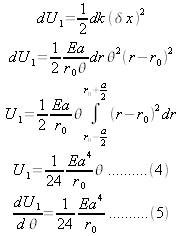If a buckling happens after enough twisting, the energy required to form a circular writhe with a radius r0 :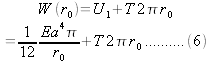This energy cost is minimum at a certain radius. It is well-known that the geometric mean of the numbers is always less then the arithmetic mean of them. Therefore,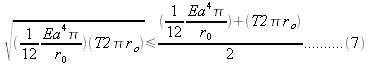Then the minimum required energy: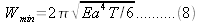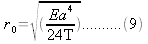The torsional energy of a twisted elastic rod is U2(φ)=½κ φ2, where φ=2nπ the twisted angle and κ is the torsional coefficient.

At the critical torque value, the work done by twisting the rod by one rotation, must be equal to the conformation energy of the loop.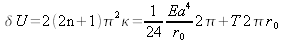So the twist number required for buckling a writhe: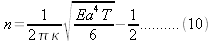This simple model explains how the two types of coiling of DNA related with each other.

© 2007 U. Eser. The author grants permission to copy, distribute and display this work in unaltered form, with attribution to the author, for noncommercial purposes only. All other rights, including commercial rights, are reserved to the author.

References

T. Strick, "Twisting and Stretching Single DNA Molecules," Prog. Biophys. Mol. Bio. 74 , 115 (2000).

J. H. White, "Self Linking and the Gauss Integral in Higher Dimensions," Am. J. Math. 91, 693 (1969).